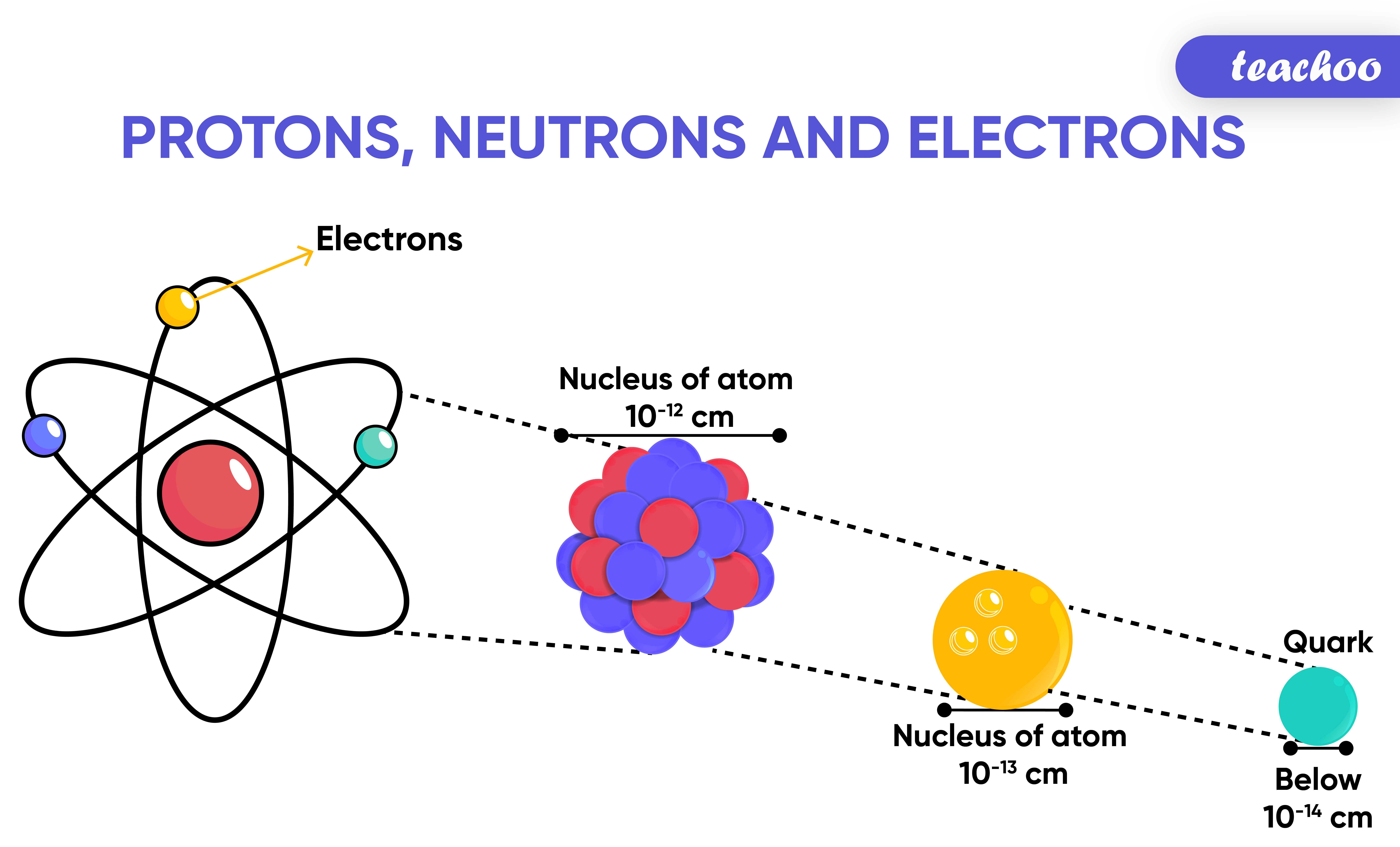## What are Nucleons?

We know that every atom contains protons,neutrons and electrons

Out of this

Protons and Nucleons are present in nucleus of atom

They are together called nucleons## What is a Molecule?

A group of atoms chemically bonded together is Here are the valency of a few elements:

called Molecule.

## What is atomic number?

It is the number of protons present in the nucleus of an atom

It is denoted by Z

Example 1

For Hydrogen, Z = 1

It means that in hydrogen atom, there is 1 proton present in the nucleus

Example 2

For Carbon, Z=6

It means that in carbon atom, there are 6 protons in the nucleus

## What is a Molecule?

A group of atoms chemically bonded together is Here are the valency of a few elements:

called Molecule.

## What is Mass Number?

It is the total number of protons and neutrons present in nucleus of atom

It is denoted by A.

Example 1

Carbon atom has 6 protons and 6 neutrons

So, Mass of Carbon is 6+6 = 12 u

Example 2

Aluminium atom has 13 protons and 14 neutrons

So Mass of Aluminium = 13+14 = 27 u

## What is a Molecule?

A group of atoms chemically bonded together is Here are the valency of a few elements:

called Molecule.

1. Class 9
2. Chapter 3 Class 9 - Atoms And Molecules (Term 2)
3. Concepts

Concepts

Class 9
Chapter 3 Class 9 - Atoms And Molecules (Term 2)